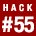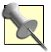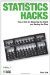# Hack 55. Predict the Game WinnersThe information provided by correlations allows for predicting any outcome, especially sports. With multiple regression techniques and a little software, you can guess the winner before the game is played. The trick is picking the right predictors.

The conventional use of correlations [Hack #11] is to find out how much two variables share in commonor, more technically, how much variance is shared between the two variables.Shared variance is a mathematical term to describe the amount of redundant information reflected in two variables. When lots of variance is shared, prediction is easy and accurate because knowledge of one variable leads to knowledge about a second. Shared variance is estimated by squaring the correlation.

But our everyday world consists of way more than only one variable predicting another. In fact, in most cases there are several or multiple variables that predict a particular outcome. Here we are not dealing with the prediction of just one variable from another, but the prediction of one variable from several. This tool is called multiple regression (because there is more than one predictor variable).

Serious sports gamblers, bookies, and casino operators are familiar with multiple regression, or at least they should be. So much information is available about sports teams that there are almost certainly all sorts of variables that, in the right combinations, can fairly accurately predict which team will win.

Betting on professional football is one of the most common of all gambling practices (or so I have been told). This hack shows how to gather data and use multiple regression to predict the winner of any football match up. This example involves predicting who will win the Super Bowl, the National Football League's championship game.

#### Choosing Predictor Variables

The first step is to build your model (the predictors and their weights that you will use to make your prediction). For football, there are dozens of statistics kept and available about teams' past performances and player characteristics. Some make sense as predictors of future performance (e.g., past performance), while others do not (e.g., cuteness of the mascot). The chance to win money, though, is a powerful motivator, so I would take the time and effort to collect just about every statistic I could find about every team and every game. The key is to find variables that on their own correlate pretty well with winning the Super Bowl.

Let's pretend that you have done your research and found six variables that correlate with whether a team wins or loses. Some make sense; some do not. You are interested in getting the most accurate real-life prediction you can get, so you are willing to include the kitchen sink if it will make a difference. To be clear, you took each year that a team was in a Super Bowl and then gathered data for that team from that year.

Imagine you've found that the following variables are of interest and might be useful in predicting this outcome based on previous years' performance and the characteristics of 30 teams. The variables you'll be using in your model begin with the outcome of interestnamely, did the team win the Super Bowl during the year that the data is gathered from (Yes = 1, No = 2)?

The following variables were found to correlate with the outcome:

• Number of easy wins during the season (won by more than nine points)

• Average attendance during the season

• Average number of hot dogs sold per game

• Average temperature of team's Gatorade

• Average weight of defensive linemen

When you do this analysis with real data, you'll likely find a different mix of potential predictors.

#### Entering the Data into a Spreadsheet

Social scientists often use statistical software such as SPSS or SAS, but for this example, I used an Excel worksheet and Excel's very cool Data Analysis Toolpack (and the Regression Tool). I entered some made-up but realistic data into the spreadsheet shown in Table 5-10.What? You thought I was going to show you a real secret formula for predicting the outcomes of football games? I'm only showing you how to make your own. I'll keep mine to myself, thank you very much!

##### Table Super Bowl predictors
A11156,5334,79856276
B2944,543571576311
C1845,5439,75345315
D1645,7688,02046311
E1876,7865,39556256
F11156,5331,05467277
G2956,55475076256
H21244,6756,57677254
I21156,6679,18777287
J21065,5454,53387301
K21278,7561,96386243

Table 5-10 shows some of the 30 rows of fictional data I collected, representing 30 examples I used in my statistical analysis. The more rows of data, the more instances you can get and the more accurate your eventual predictions will be.

#### Building a Regression Equation

You might remember from your high school days that the formula for a simple straight line looks something like this:

This equation is made up of the following variables:

Y'

Predicted score on variable Y

b

The slope of the line

X

The score of a single predictor

a

The intercept (where the straight line crosses the Y or vertical axis)

So, for example, if you wanted to predict human height from weight and had a bunch of data to create such a formula after plugging in the various values, you might get something that looks like this:

This means that if your weight (the X variable) is 125 pounds, the prediction is that you will be about 64 inches tall, or about 5 feet 3 inches.

But when we have more than one predictor variable, things get more interesting and more fun. There is a longer series of predictors (many Xs) and weights (many bs).

I ran a multiple regression analysis using this data in SPSS, a statistical software program, but you can get much of the same information using Excel (see the "Getting Regression Info in Excel" sidebar).

## Getting Regression Info in Excel

There are two ways to get statistical regression info using Excel. First, you can use the SLOPE and INTERCEPT functions, which you can find on the InsertFunction menu. Select the function and enter the argument (the cells where the data is located), and Excel returns these values, allowing you to plug in known values and predict others. This method works best when there is just one predictor.

You can also make use of the Regression option in the Data Analysis ToolPak, an Excel add-on (which you might have to install). Using this option on the Tools menu, you can test the significance of the regression coefficient using an F test, a statistical test similar to a t test [Hack #17].

The results (a.k.a. the output) are shown in Tables 5-11 and 5-12. Let's see which of the variables best assist us in predicting whether a team will win the Super Bowl.

##### Table Regression statistics
Multiple R R square Observations
0.84830.719630

##### Table Regression equation
VariableCoefficientsT statP-value
Intercept-0.784-1.0100.323
Easy wins0.1194.2740.000
Attendance0.000-0.8220.416
Hot dogs sold0.0001.0430.308
Weight0.0010.5800.567

Table 5-12 shows a coefficient (a weight) for each of the five variables that were entered into the equation to test how well each one predicts Super Bowl wins. For example, the coefficient associated with "Easy wins" is .119.

If we combine all of these into one big equation for predicting Super Bowl outcomes, here's the model we get:

So, for each of the predictors (variables X1 through X5), there is specific weight (the bs in the formula or the coefficients in the results).

Now, the same formula in English:

b*Wins + b*Average Attendance + b*Hot Dogs + b*Temp + b*Weight + a

And using the numbers from the output shown in Table 5-12, here's the real live regression equation:

#### Interpreting and Applying the Regression Equation

Imagine using this equation with all the rows of data you entered into your spreadsheet. There would be a pretty high correlation between the actual Super Bowl outcomes and the predicted outcome. I know this because of the "Multiple R" part of the output shown in Table 5-11, which shows a pretty high correlation. 0.84 is close to 1, which is the highest correlation you could get.The "R square" of .72 is the proportion of shared variance that we talked about earlier in this hack.

What does this mean? The combination of these predictor variables is a pretty effective way to judge whether a team will win the Super Bowl. Foolproof? Of course not, since the combination of these variables does not perfectly predict the outcome, but it does a pretty solid job.

So, let's say that this year's Denver Cannonballs has the data points shown in Table 5-13.

##### Table Data for Denver Cannonballs
VariableValue
Easy wins13
Attendance35,678
Hot dogs4,567
Weight267

Plugging this data into the equation shown earlier, here's what we get for a predictor of Y:

The final value for Y is 1.875, a bit closer to 2 (meaning they are not predicted to win) than to 1 (meaning they are predicted to win).

What's the key to a good set of predictors?

• All the predictors should be independent of each other (if at all possible) since you want them to make a unique contribution to the understanding of what you are predicting.

• Each of the predictors should be as highly related as possible to the outcome that you are predicting.

A careful examination of the equation produced in this hack indicates that the bulk of the predictive power comes from just two variables: the number of easy victories and the temperature of the team's Gatorade. Also, many of the predictors have zero weights, which means you don't need them at all. You could remove these unhelpful variables (attendance and hot dogs sold) to streamline your formula. In fact, collecting data on easy wins and Gatorade temperature alone is enough to make fairly accurate predictions in our example.

Neil SalkindStatistics Hacks: Tips & Tools for Measuring the World and Beating the Odds
ISBN: 0596101643
EAN: 2147483647
Year: 2004
Pages: 114
Authors: Bruce Frey

Similar book on Amazon